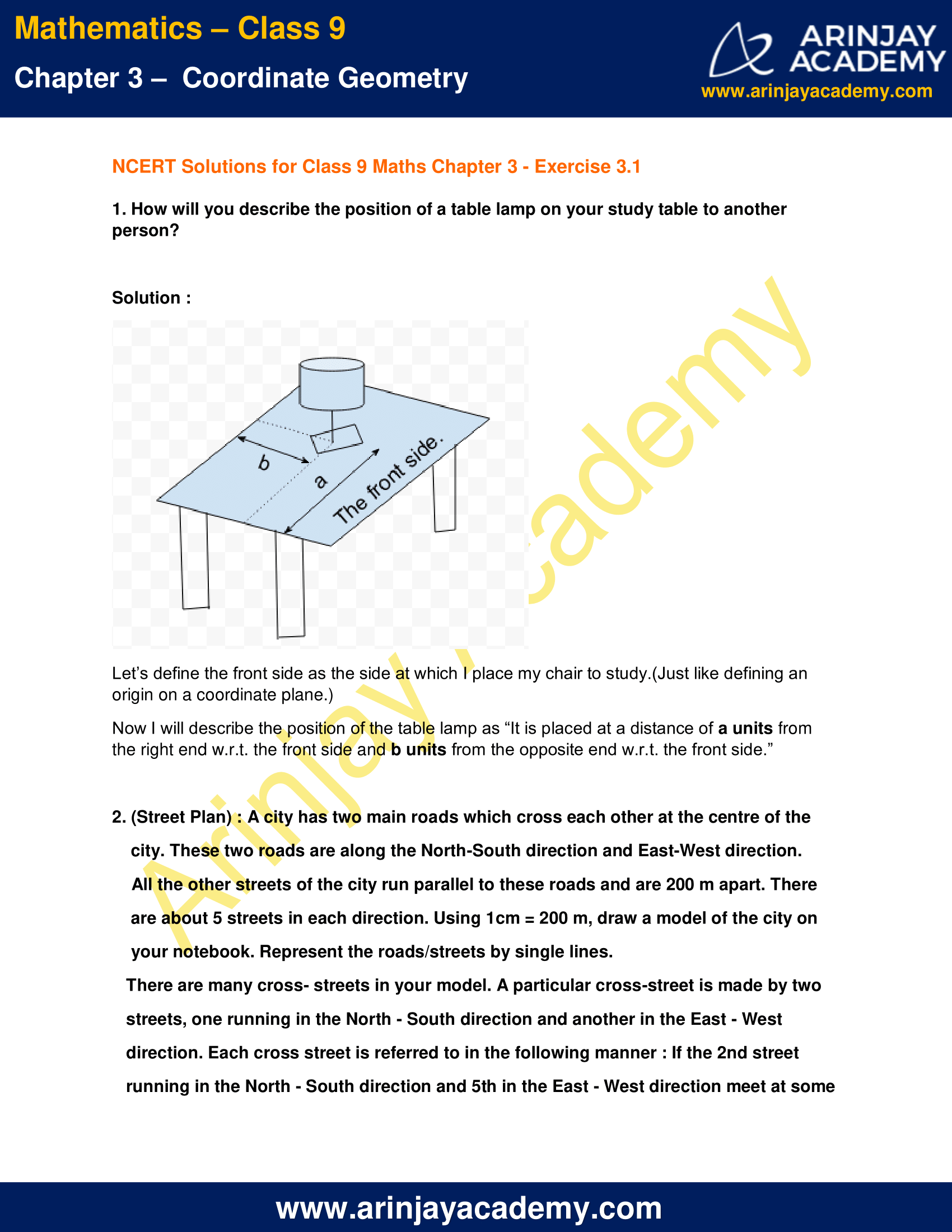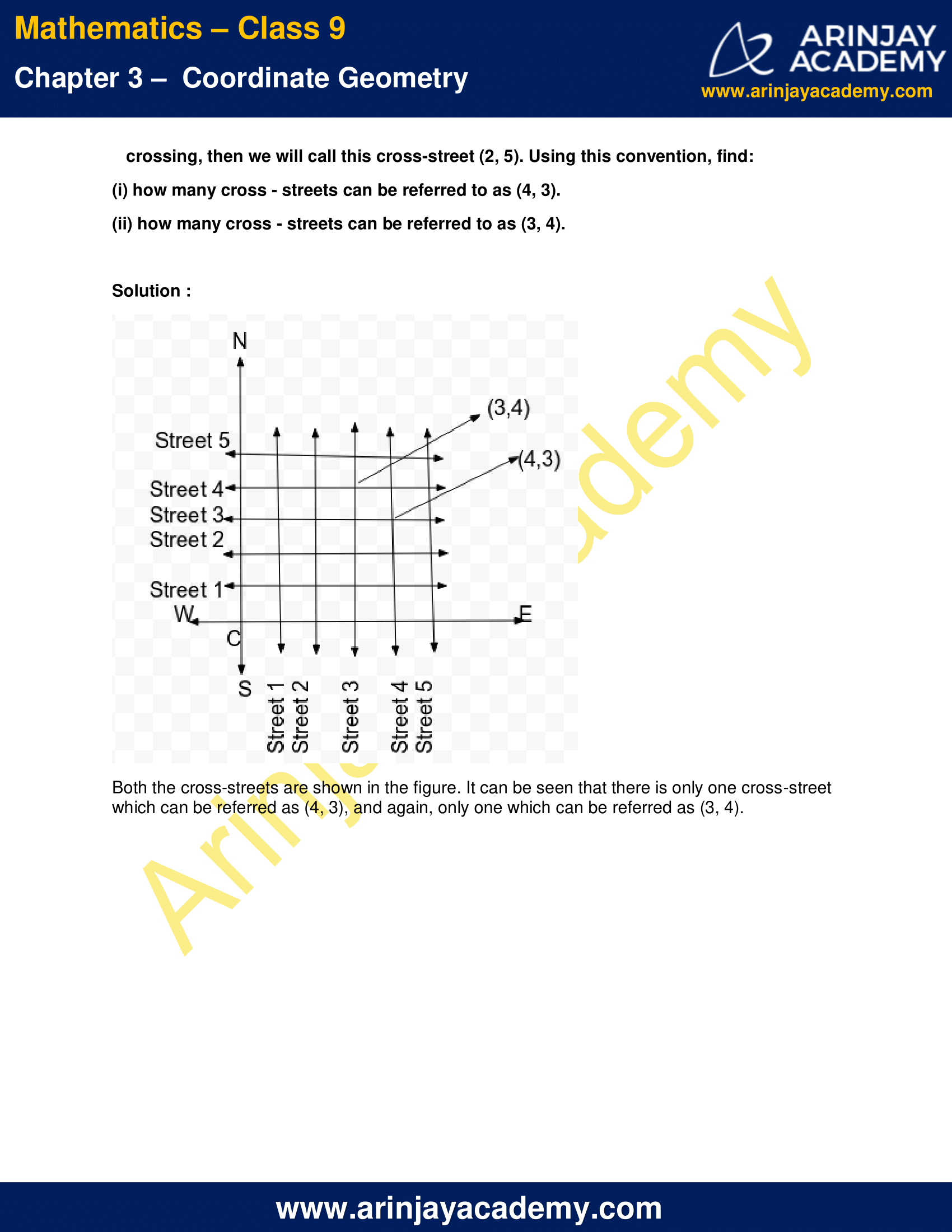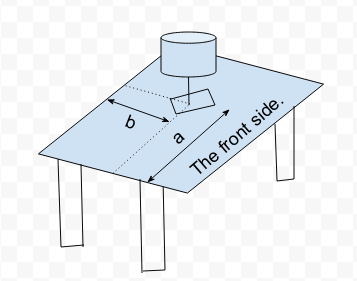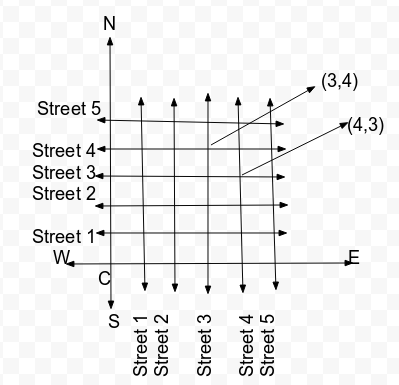# NCERT Solutions for Class 9 Maths Chapter 3 Exercise 3.1 – Coordinate Geometry

Download NCERT Solutions for Class 9 Maths Chapter 3 Exercise 3.1 – Coordinate Geometry. This Exercise contains 2 questions, for which detailed answers have been provided in this note. In case you are looking at studying the remaining Exercise for Class 9 for Maths NCERT solutions for Chapter 3 or other Chapters, you can click the link at the end of this Note.

### NCERT Solutions for Class 9 Maths Chapter 3 Exercise 3.1 – Coordinate GeometryNCERT Solutions for Class 9 Maths Chapter 3 Exercise 3.1 – Coordinate Geometry

1. How will you describe the position of a table lamp on your study table to another person?

Solution :Let’s define the front side as the side at which I place my chair to study.(Just like defining an origin on a coordinate plane.)

Now I will describe the position of the table lamp as “It is placed at a distance of a units from the right end w.r.t. the front side and b units from the opposite end w.r.t. the front side.”

2. (Street Plan) : A city has two main roads which cross each other at the centre of the city. These two roads are along the North-South direction and East-West direction. All the other streets of the city run parallel to these roads and are 200 m apart. There are about 5 streets in each direction. Using 1cm = 200 m, draw a model of the city on your notebook. Represent the roads/streets by single lines.
There are many cross- streets in your model. A particular cross-street is made by two streets, one running in the North – South direction and another in the East – West direction. Each cross street is referred to in the following manner : If the 2nd street running in the North – South direction and 5th in the East – West direction meet at some crossing, then we will call this cross-street (2, 5). Using this convention, find:

(i) how many cross – streets can be referred to as (4, 3).
(ii) how many cross – streets can be referred to as (3, 4).

Solution :Both the cross-streets are shown in the figure. It can be seen that there is only one cross-street which can be referred as (4, 3), and again, only one which can be referred as (3, 4).

NCERT Solutions for Class 9 Maths Chapter 3 Exercise 3.1 – Coordinate Geometry, has been designed by the NCERT to test the knowledge of the student on the topic -Introduction of Coordinate Geometry

The next Exercise for NCERT Solutions for Class 9 Maths Chapter 3 Exercise 3.2 – Coordinate Geometry can be accessed by clicking here.